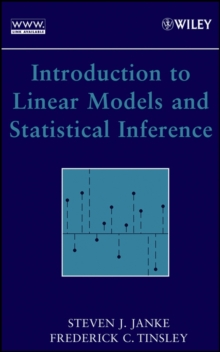Supporting your high street Find out how »
• My AccountIntroduction to Linear Models and Statistical Inference Hardback

Description

This is a multidisciplinary approach that emphasizes learning by analyzing real-world data sets.

This book is the result of the authors' hands-on classroom experience and is tailored to reflect how students best learn to analyze linear relationships.

The text begins with the introduction of four simple examples of actual data sets.

These examples are developed and analyzed throughout the text, and more complicated examples of data sets are introduced along the way.

Taking a multidisciplinary approach, the book traces the conclusion of the analyses of data sets taken from geology, biology, economics, psychology, education, sociology, and environmental science.

As students learn to analyze the data sets, they master increasingly sophisticated linear modeling techniques, including: Simple linear models; Multivariate models; Model building; Analysis of variance (ANOVA); Analysis of covariance (ANCOVA); Logistic regression; and, total least squares.

The basics of statistical analysis are developed and emphasized, particularly in testing the assumptions and drawing inferences from linear models. Exercises are included at the end of each chapter to test students' skills before moving on to more advanced techniques and models.

These exercises are marked to indicate whether calculus, linear algebra, or computer skills are needed.

Unlike other texts in the field, the mathematics underlying the models is carefully explained and accessible to students who may not have any background in calculus or linear algebra.

Most chapters include an optional final section on linear algebra for students interested in developing a deeper understanding.

The many data sets that appear in the text are available on the book's Web site.

The MINITAB[registered] software program is used to illustrate many of the examples.

For students unfamiliar with MINITAB[registered], an appendix introduces the key features needed to study linear models.

With its multidisciplinary approach and use of real-world data sets that bring the subject alive, this is an excellent introduction to linear models for students in any of the natural or social sciences.

Information

• Format: Hardback
• Pages: 600 pages
• Publisher: John Wiley & Sons Inc
• Publication Date:
• Category: Mathematics
• ISBN: 9780471662594

£132.00

£117.05

on all orders

Pick up orders

from local bookshops

£55.50

£47.55